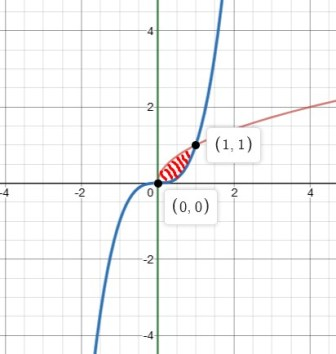# Evaluate the volume of revolution formed by revolving the region bounded by y = x^{1 / 2} and y =...

## Question:

Evaluate the volume of revolution formed by revolving the region bounded by {eq}\displaystyle y = x^{\dfrac 1 2} {/eq} and {eq}y = x^3 {/eq} around y axis.

## Method of shells:

a) If the axis of revolution is horizontal, then: {eq}V=2\pi\int_a^bp(y)f(y)dy {/eq} where {eq}p(y) {/eq} is the distance between the axis of revolution and the {eq}x {/eq}-axis. If the curve rotates around the {eq}x {/eq}-axis: {eq}V=2\pi\int_a^byf(y)dy {/eq}.

b) If the axis of revolution is vertical, then:{eq}V=2\pi\int_a^bp(x)f(x)dx {/eq} where {eq}p(x) {/eq} is the distance between the axis of revolution and the {eq}y {/eq}-axis. If the curve rotates around the {eq}y {/eq}-axis: {eq}V=2\pi\int_a^bxf(x)dx {/eq}.

## Answer and Explanation:

Consider the curves

{eq}\displaystyle y = x^{\frac{1}{2}}, \, y = x^3, \: \: \, \text{about the y-axis that is} \,\: x=0 {/eq}

The point of intersection of the two curves is as follows

{eq}\displaystyle x^{\frac{1}{2}}=x^3\Rightarrow x=x^6\Rightarrow x(x^{5}-1)=0\\ \displaystyle \Rightarrow x=0,\:x=1 {/eq}

Therefore, the point of intersection are (0,0) and (1,1)The volume of the curves about x=0 given by shell method is as follows

{eq}\displaystyle \text{Volume}=2\pi\int_{a}^{b}r\: h\: dx\\ \displaystyle =2\pi\int_{0}^{1}(x-0)\:(x^{\frac{1}{2}}-x^3) \: dx\\ \displaystyle =2\pi\int_{0}^{1} (x^{\frac{3}{2}}-x^4) \: dx\\ \displaystyle =2\pi\left ( \frac{2}{5} x^{\frac{5}{2}}-\frac{1}{5}x^5\right )_{0}^{1}\\ \displaystyle =2\pi\left ( \frac{2}{5} x^{\frac{5}{2}}-\frac{1}{5}x^5\right )_{0}^{1}\\ \displaystyle =2\pi\left ( \frac{2}{5} -\frac{1}{5} \right )\\ \displaystyle =2\pi\times \frac{1}{5}\\ \displaystyle =\frac{2\pi }{5} {/eq}

#### Learn more about this topic:What is Volume in Science? - Lesson for Kids

from Science for Kids

Chapter 1 / Lesson 14
64K